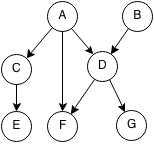## Introduction

Prerequisites: Graph Theory, Depth First Search

A topological sort or topological order of a directed graph is an order in which every node comes after its ancestors.For example topological orders could be:

• (A, B, C, D, E, F, G)
• (B, A, D, C, F, E, G)
• (B, A, D, G, F, C, E)

But (B, A, C, F, D, E, G) is not a topological ordering because D is an ancestor of F and it comes after F.

## Implementation

Topological sort can implemented in O(n) time using DFS for a directed acyclic graph (a digraph with no cycles). How it works:

1. Start with an empty top order.
2. Pick any unmarked node.
3. Get the DFS preordering from that node for unvisited nodes.
4. Add the DFS to the head of the current order.
5. Mark every node that has been visited.Example:

• Pick C
• DFS preorder from C is (C,E)
• Add DFS preorder to head [C,E]
• Pick F
• DFS preorder from F is (F)
• Add DFS preorder from F to head [F,C,E]
• Pick B
• DFS preorder from B is (B,D,G)
• Add DFS preorder from B to head [B,D,G,F,C,E]
• Pick A
• DFS preorder from A is (A)
• Add DFS preorder from A to head [A,B,D,G,F,C,E]
• Done, all nodes visited

A DFS order from a node is guaranteed to be a topological order. Since we add everything to the head of the order, a child of a node cannot appear before it.